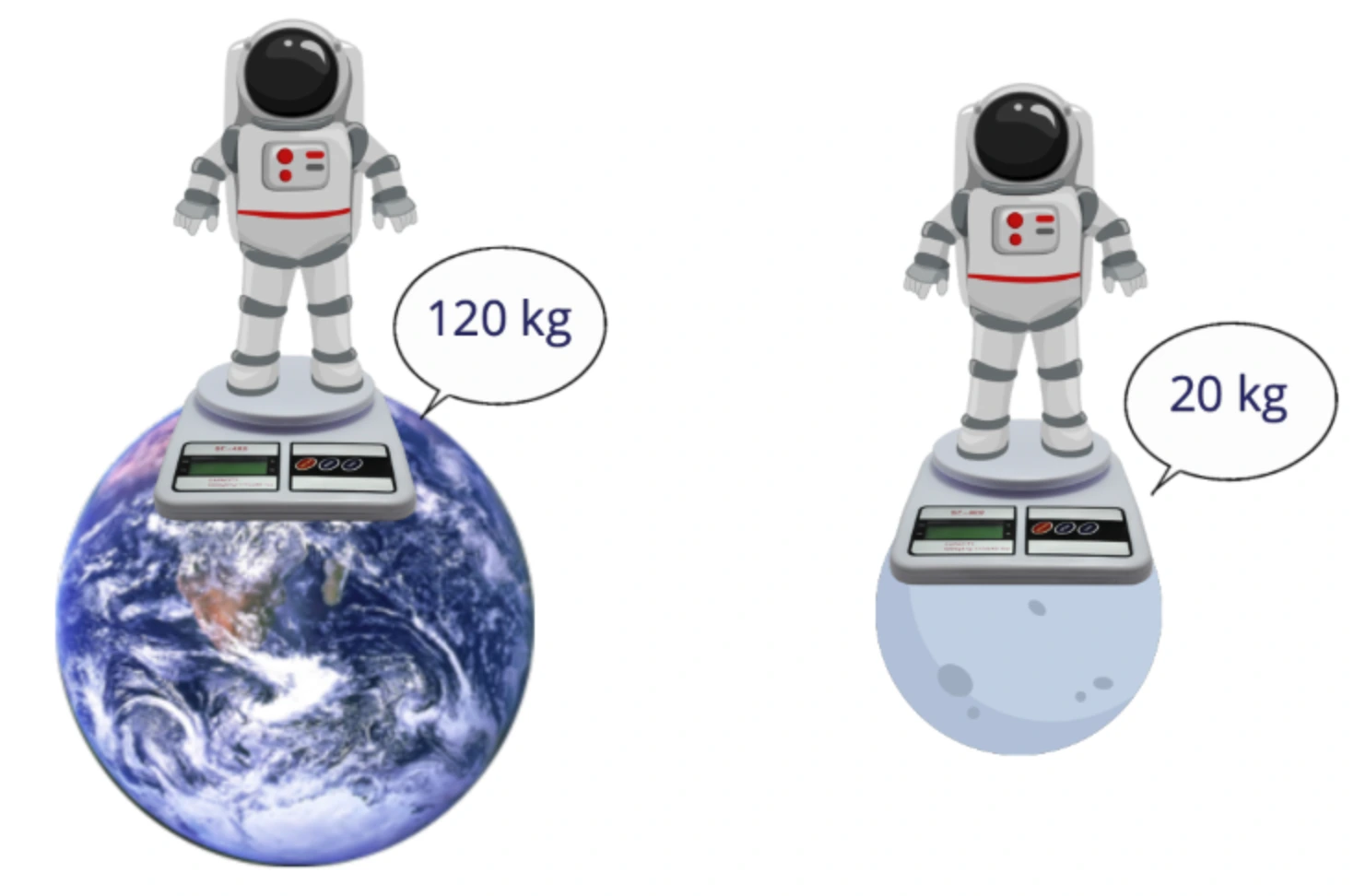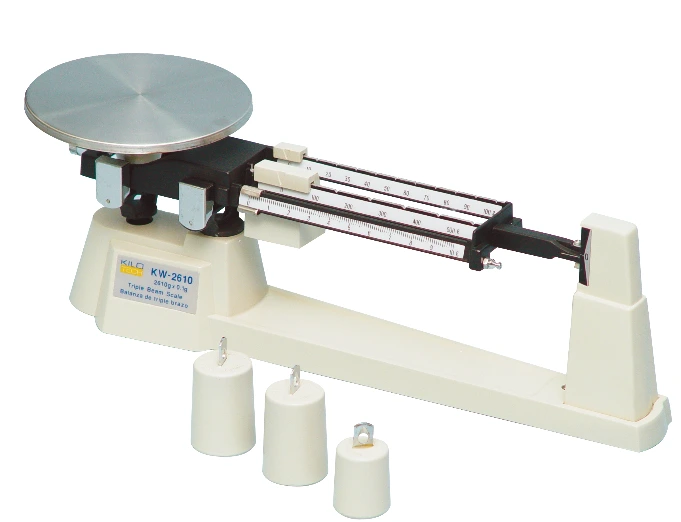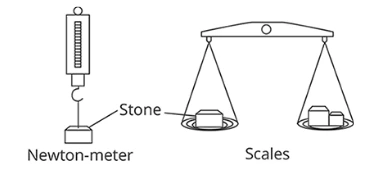Study S3 Physics Mass, Weight & Density - Geniebook# Mass, Weight & Density

• Mass
• Weight
• Inertia

## Mass & Weight

Mass is the amount of matter in a body. The SI unit of mass is the kilogram (kg).

The weight of an object is the amount of gravitational force acting on it. The SI unit of weight is the Newton (N).

## Gravitational Field

A gravitational field is a region in which a mass experiences a force due to gravitational attraction.

When a mass is placed in a gravitational field, it experiences a gravitational force. This gravitational force is also known as weight.

### Gravitational Field Strength

Gravitational field strength is defined as the gravitational force acting per unit mass. It represents how strong the gravitational field is. On Earth, the gravitational field strength is approximately $$10 \text{ Nkg}^{-1}.$$

In Kinematics, we have studied acceleration due to gravity. This acceleration is due to the gravitational force. The value of the acceleration due to gravity is as follows:

$$\textbf{Acceleration due to gravity } = 10 \text{ ms}^{-2}$$

The gravitational field strength is the same as the acceleration due to gravity. Very often, the units can be used interchangeably in questions.

Let us see how both the units are the same.

$$10 \text{ Nkg}^{-1} \qquad 10 \text{ ms}^{-2}$$

We know,

$$\textbf{F } = \textbf{m } \times \textbf{ a}$$

Let us put the units of the different elements.

$$\text{N } = \text{ kg} × \text{ ms}^{-2}$$

Let us substitute N above

$$\text{Nkg}^{-1} = \text{kg} \times \text{ms}^{-2} \times \text{kg}^{-1}$$

$$\text{Nkg}^{-1} = \text{ms}^{-2}$$

Thus,

$$10 \text{ Nkg}^{-1} ≡ 10 \text{ ms}^{-2}$$

Hence, we see that gravitational friend strength and the acceleration due to gravity are the same. Both units are equivalent and hence can be used interchangeably.

### Earth’s Gravitational Field

The strength of Earth’s gravitational field is strongest at the surface of Earth and gets weaker farther away.

The gravitational force experienced by an object (weight) due to the Earth’s gravitational field gets stronger as the object moves closer to Earth.

## Mass & Weight

Mass

Weight

The amount of matter in a body

A gravitational force

A scalar quantity

A vector quantity

SI Unit : kg

SI Unit : N

Independent of gravitational field strength

Dependent on gravitational field strength

Since the weight $$\textit{W}$$ of an object is the gravitational force acting on it, and the acceleration due to gravity is equal to the gravitational field strength $$\textit{g}$$,

By Newton’s Second Law of Motion,

$$\textbf{F } = \textbf{m } \times \textbf{ a}$$

where,

$$\textit{F}$$ is the resultant force

$$\textit{m}$$ is the mass of object

$$\textit{a}$$ is the acceleration experienced by the object in motion

Replacing $$\textit{F}$$ with weight $$\textit{W}$$ , and $$\textit{a}$$ with the acceleration due to free fall $$\textit{g}$$, we get

$$\textbf{W} = \textbf{mg}$$

This is the relationship between mass and weight.

We see from the above formula that weight $$\textit{W}$$ is dependent on gravity $$\textit{g}$$. The higher the gravitational field strength, the larger is the weight of an object. The mass of the object remains the same as it is independent of the gravitational field strength, $$\textit{g}$$.

### Practice Questions

Question 1:

How does the mass and weight of Albert change as he travels from outer space to Earth?

Mass Weight
(A) Decreases Decreases
(B) Decreases No Change
(C) No Change Increases
(D) Increases Increases

Solution:

Option C is the correct answer.

Explanation:

The mass of the object does not change.
As the object comes closer to Earth from space, the gravitational force acting on the object increases and thus the weight of the object increases as it comes closer to Earth.

Question 2:

An 15 kg object on the Earth is brought to the Moon. The gravitational field strength on the Earth is 10 Nkg$$^{-1}$$ and on the Moon is 1.6 Nkg$$^{-1}$$. What is the mass and the weight of the object on the Moon?

Mass / kg Weight / N
(A) 15 24
(B) 15 150
(C) 24 15
(D) 150 24

Solution:

Option A is the correct answer

Explanation:

We learnt that the mass of the object does not change. So the mass of the object on earth as well as the moon remains the same.

Mass of the object $$\text{m } = 15 \text{ kg}$$

$$\textbf{W} = \textbf{mg}$$

Gravitational force on the moon, $$\text{g } = 1.6 \text{ Nkg}^{-1}$$

\begin{align} \textbf{W } &= 15 \times 1.6 \\[2ex] &= 24 \text{ N} \end{align}

## Measuring Mass & Weight

Common weighing instruments such as the electronic balance and spring balance actually measure the weight of an object and not its mass.

They are calibrated to give mass readings according to Earth’s gravitational field strength.

When used at places with different gravitational field strength, they will give inaccurate readings.

Earth                                            Moon

######Image Credits: hiclipart.com

How do we measure the mass of an object?

The mass of an object does not depend on the gravitational field strength.

It can be measured by using a beam balance.

######Image Credits: hiclipart.com

The beam balance compares the gravitational force acting on an object with standard masses.

Since both the object and the standard masses experience the same gravitational field strength, the mass readings obtained on the Earth and on the Moon will be the same.

Question 3:

A lump of stone is weighed using a Newton-meter (spring balance) and a pair of scales (beam balance). This experiment is repeated on the Moon.

######Image credits: GenieSmart

Are the readings for the Newton-meter and the scales the same when taken on the Earth and on the Moon?

On Newton - Meter On Scales
(A) Different Different
(B) Different Same
(C) Same Different
(D) Same Same

Solution:

Option B is the correct answer

Explanation:

The Newton-meter (spring balance) measures the weight of the object. Since the weight is dependent on the gravitational field strength so it will change when taken on  Earth and on the Moon.
The gravitational field strength of Earth is more than the gravitational field strength of the Moon. So  the weight of the object on the Earth and on the Moon will be different.
The scales (beam balance) measure the mass of the object. Since the mass of an object is independent of the gravitational field strength, the mass of the object on the Earth and on the Moon will be the same.

## Newton’s 1st Law Of Motion & Inertia

Newton’s First Law of Motion states that an object will continue in its state of rest or uniform motion in a straight line unless a resultant force acts on it. The inertia of an object refers to the reluctance of the object to change its state of motion due to its mass.

Newton's First Law of Motion implies that a stationary object tends to stay at rest, and that a moving object tends to continue in its state of motion.

Thus, Newton’s First Law of Motion is also called the law of inertia.

## Inertia

Mass is a measure of inertia. The greater the mass of an object, the greater is its inertia.

Let us see the way inertia plays out.

If you are chased by an elephant, how should you run? Will you run as fast as you can in a straight line?

In that case, do you think you will be able to beat the elephant? The chances are NO. The elephant will certainly be able to catch you.

But, what if you run in a zig-zag manner ? Will the elephant still be able to catch you?

If you run in a zig-zag manner and change your direction frequently, you have a high chance that you will escape unhurt. Why ?

The elephant has more mass and so more inertia. According to the Law of Inertia, the body continues to be at rest or move uniformly in a straight line unless a resultant force is acted on it.

Hence it will be difficult for the elephant to change directions frequently to chase you. But since you have less mass, it will be much easier for you to change direction and run.

### Inertia In Everyday Life

Inertia can be observed in everyday life. Observe what happens to the passengers in the MRT train when the train suddenly brakes. The passengers will tend to continue moving forward when the train brakes and may fall down in the process.

This phenomenon is due to inertia.

### Inertia & gravity

Inertia is dependent only on mass. It is not affected by the gravitational field strength. An object will have the same inertia on the Moon as it does on Earth, despite the fact that gravitational field strength on the Moon is much weaker.

Question 4:

The diagram below shows a container which is filled with water. It is placed on a frictionless surface with a piece of smooth paper beneath it. When the paper is quickly pulled away from the bottom of the container,it remains stationary.

######Image credits: GenieSmart

Which property of the container causes it to remain stationary?

1. weight
2. friction
3. air resistance
4. inertia

Solution:

Option D is the correct answer

Explanation:

We know that the container filled with water has more mass and thus more inertia. So, when the smooth paper is pulled away quickly, the container remains stationary due to inertia. As per the Law of Inertia, the container will continue to be at rest.

## Conclusion

I hope you found the article interesting and you were able to clarify your concepts around mass, weight, inertia, gravitational field as well as Newton’s 1st law of motion and inertia.

Continue Learning
Light - Reflection Units and Measurements
Kinematics - Distance & Displacement Dynamics
Mass, Weight & Density Thermal Properties Of Matter
Moments Temperature
Kinetic Model of Matter Waves
Work, Power & Energy Pressure
Transfer Of Thermal EnergyPrimaryPrimary 1Primary 2Primary 3Primary 4Primary 5Primary 6SecondarySecondary 1Secondary 2Secondary 3Physics
Light - Reflection
Units And Measurements
Kinematics - Distance & Displacement
Dynamics
Mass, Weight & Density
Thermal Properties Of Matter
Moments
Temperature
Kinetic Model of Matter
Waves
Work, Power & Energy
Pressure
Transfer Of Thermal EnergyChemistryMathsSecondary 4